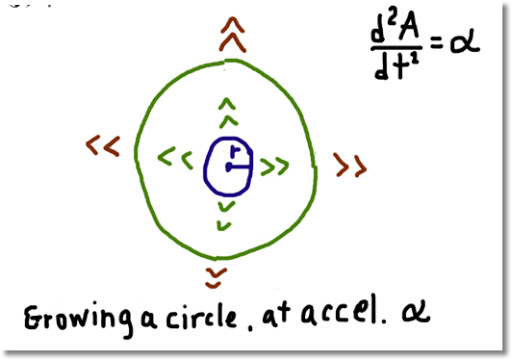## Monday, January 7, 2013

### Growing a Circle at an Accelerated RateConsider a circle. The circle starts as a dot and grows in size by α square units (per time unit). Can we determine when the size of a circle grows to a certain point?

It turns out, we can.

Let A(t) describe the area of the circle at time t. The instantaneous change of the area can be determined by taking the derivative, or A'(t).

The speed of the circle's growth is changing. We determine the speed of the growth by taking the second derivative, A''(t).

In the beginning, the circle starts out as an dot with virtually no area, but has an accelerated growth of α.

(I) A''(t) = α
with initial conditions A(0) = 0 and A'(0) = 0.

Taking the integral of (I):

∫ A''(t) dt = ∫ α dt

A'(t) = α * t + C

With A'(0) = 0,

0 = α * 0 + C,

and C = 0. Hence:

(II) A'(t) = α * t

Applying the integral again, and taking the initial conditions into account:

(III) A(t) = 1/2 * α * t^2

Knowing that the area of a circle with radius r is A = π * r^2; we can relate the circle's radius to the circle's growth by:

(IV) π * r^2 = 1/2 * α * t^2

Examples
1. If a circle grows an accelerated rate of α = 0.1 in^2/s^2, how long will it take for the circle's area to grow to 10 in^2?

With α = 0.1 and A = 10:

10 = 1/2 * (0.1) * t^2

t^2 = √200

t ≈ 14.14214

It takes just over 14 seconds for the circle to grow to 10 square inches.

2. If a circle, from a virtual dot, takes 10 minutes to grow a size where it has radius of 25 inches, determine the its accelerated growth.

The area of a circle at 10 minutes is A(10) = π * 25^2 ≈ 1963.49541 in^2. Then:

A(10) = 1/2 * 10^2 * α

π * 25^2 = 50 * α

α ≈ 39.26991

The acceleration of the circle's growth is about 39.27 in^2/min^2.

Until next time,

Eddie

This blog is property of Edward Shore. 2013

### Casio fx-CG50: Sparse Matrix Builder

Casio fx-CG50: Sparse Matrix Builder Introduction The programs can create a sparse matrix, a matrix where most of the entries have zero valu...